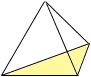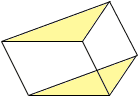Subject: Geometry Definitions What's the definition of a Triangular Prism and a Triangular pyramid. Tyler 4th grade Hi Tyler, A triangular pyramid is a geometric solid with a base that is a triangle and all other faces are triangles with a common vertex.Triangular pyramid A triangular prism is ageometric solid with two bases that are congruent (identical), parallel triangles and all other faces are parallelograms. It is referred to as a right triangular prism if the faces are rectangles.Triangular prism Cheers,Penny Go to Math Central